## Struggling with classes / types

General FreeBASIC programming questions.
dodicat
Posts: 5826
Joined: Jan 10, 2006 20:30
Location: Scotland

### Re: Struggling with classes / types

Dafhi.
I can't think offhand of any tidy pointer stuff I made up recently.
The latest offering I think was a million boxes via ptr.
I think you can just utilise type without the hype in 90% of cases.

A million boxes:
Press any key for another million.
toggles empty and filled.

Code: Select all

` dim shared as long lim=1000000 dim shared as long copy 'for the graphical destructor copy=lim dim as ulong black=rgb(0,0,0),white=rgb(255,255,255)  type box    as ulong ptr p =new ulong              'all the spatial information    declare sub draw()                        'drawing method    declare destructor                        'Goodbye, thanks for the memoryend typesub box.draw()    dim as ulong colour=rgba(cast(ubyte ptr,@p),cast(ubyte ptr,@p),cast(ubyte ptr,@p),cast(ubyte ptr,@p))   if p then line(p,p)-(p+p,p+p),colour,bf else _                line(p,p)-(p+p,p+p),colour,bend subdestructor boxlim-=1#define map(a,b,x,c,d) ((d)-(c))*((x)-(a))/((b)-(a))+(c)var x=map(copy,0,lim,100,700)var y=map(100,700,x,100,500)circle(x,y),20,rgb(200,0,0),,,,fcircle(x,600-y),20,rgb(200,0,0),,,,fdelete [] pend destructorscreen 19,32,,64#define range(f,l) Int(Rnd*((l+1)-(f))+(f))dim as byte q=1dim as box b(1 to lim)     do    cls    q=-q    for n as long=1 to lim        with b(n)            .p=range(100,700)         'x            .p=range(100,500)         'y            .p=rnd*20                 'width            .p=rnd*20                 'height            .p=rnd*range(black,white)             .p=1+q                    'filler            .draw        end with    next n    sleep    loop until inkey=chr(27)   `
dafhi
Posts: 1238
Joined: Jun 04, 2005 9:51

### Re: Struggling with classes / types

yep that was it. I remember it had to be in the optimized rect type thread

I'll let it brew while I work on things. great concept
paul doe
Posts: 915
Joined: Jul 25, 2017 17:22
Location: Argentina

### Re: Struggling with classes / types

badidea wrote:Now my question is, what is the best way to link the classes together to get this working?

It all depends on your understanding of what you're trying to accomplish (otherwise it is only Cargo culting):

Code: Select all

`type RandomNumberGenerator extends Object  public:    declare constructor()    declare destructor()        declare function one() as double    declare function between( byval as integer, byval as integer ) as integer      private:    m_state( 0 to 1 ) as ulongint        m_x as ulongint    m_y as ulongint        static m_invDiv as doubleend typedim as double RandomNumberGenerator.m_invDiv = 1.0 / culngint( -1 )constructor RandomNumberGenerator()  randomize()    for i as integer = 0 to ubound( m_state )    m_state( i ) = rnd() * culngint( -1 )  next    for i as integer = 1 to 100    one()  next  end constructordestructor RandomNumberGenerator()end destructorfunction RandomNumberGenerator.one() as double  m_x = m_state( 0 )  m_y = m_state( 1 )    m_state( 0 ) = m_y  m_x xor= m_x shl 23  m_state( 1 ) = m_x xor m_y xor ( m_x shr 17 ) xor ( m_y shr 26 )    return( ( m_state( 1 ) + m_y ) * m_invDiv )end functionfunction RandomNumberGenerator.between( byval first as integer, byval last as integer ) as integer  return( int( one() * ( last + 1 - first ) + first ) )end functiontype Application extends Object  public:    declare constructor()    declare constructor( byref as Application )    declare destructor()        declare function begin() byref as Application    declare function inWindow( byval as integer, byval as integer ) byref as Application    declare function withTitle( byref as const string ) byref as Application      private:    declare operator let( byref as Application )        static m_title as stringend typedim as string Application.m_titleconstructor Application()end constructorconstructor Application( byref rhs as Application )end constructordestructor Application()end destructoroperator Application.let( byref rhs as Application )end operatorfunction Application.begin() byref as Application  return( this )end functionfunction Application.inWindow( byval w as integer, byval h as integer ) byref as Application  screenRes( w, h, 32 )  return( this )end functionfunction Application.withTitle( byref title as const string ) byref as Application  m_title = title  windowTitle( m_title )  return( this )end functiontype RGBAColor as ulongtype Rectangle extends Object  public:    declare virtual destructor()    declare virtual function draw() byref as Rectangle    declare function withPosition( byval as integer, byval as integer ) byref as Rectangle    declare function withSize( byval as integer, byval as integer ) byref as Rectangle    declare function withColor( byval as RGBAColor ) byref as Rectangle      protected:    static m_x as integer    static m_y as integer    static m_w as integer    static m_h as integer    static m_color as RGBAColorend typedim as integer Rectangle.m_x = 0dim as integer Rectangle.m_y = 0dim as integer Rectangle.m_w = 0dim as integer Rectangle.m_h = 0dim as RGBAColor Rectangle.m_color = 0destructor Rectangle()end destructorfunction Rectangle.withPosition( byval x as integer, byval y as integer ) byref as Rectangle  m_x = x : m_y = y  return( this )end functionfunction Rectangle.withSize( byval w as integer, byval h as integer ) byref as Rectangle  m_w = w : m_h = h  return( this )end functionfunction Rectangle.withColor( byval aColor as RGBAColor ) byref as Rectangle  m_color = aColor  return( this )end functionfunction Rectangle.draw() byref as Rectangle  return( this )end functiontype HollowRectangle extends Rectangle  public:    declare constructor()    declare destructor() override      declare virtual function draw() byref as Rectangle overrideend typeconstructor HollowRectangle()end constructordestructor HollowRectangle()end destructorfunction HollowRectangle.draw() byref as Rectangle  line( m_x, m_y ) - ( m_x + m_w - 1, m_y + m_h - 1 ), m_color, b  return( this )end functiontype FilledRectangle extends Rectangle  public:    declare constructor()    declare destructor() override    declare virtual function draw() byref as Rectangle overrideend typeconstructor FilledRectangle()end constructordestructor FilledRectangle()end destructorfunction FilledRectangle.draw() byref as Rectangle  line( m_x, m_y ) - ( m_x + m_w - 1, m_y + m_h - 1 ), m_color, bf  return( this )end functiontype RenderLayer extends Object  public:    declare constructor()    declare destructor()        declare function shape( byref as Rectangle ) byref as RenderLayerend typeconstructor RenderLayer()end constructordestructor RenderLayer()end destructorfunction RenderLayer.shape( byref aRectangle as Rectangle ) byref as RenderLayer  aRectangle.draw()  return( this )end function'' This one should be part of an interface, but mehfunction alternateBetween( byref aShape as Rectangle, byref anotherShape as Rectangle ) byref as Rectangle  static alternation as integer    alternation xor= 1    select case as const( alternation )    case 0      return( aShape )    case 1      return( anotherShape )  end selectend function/'  Main code    This is a quick and dirty example. I omitted most interfaces for brevity.  Don't try this at home.'/var randomNumber = RandomNumberGenerator()Application() _  .inWindow( 800, 600 ) _  .withTitle( "Some fluent boxes" )var render = RenderLayer()for i as integer = 1 to 1000000  render _    .shape( _      alternateBetween( HollowRectangle(), FilledRectangle() ) _        .withColor( randomNumber.one() * &hffffff ) _ /' <-- MrSwiss suggested this one ;) '/        .withPosition( randomNumber.between( 100, 700 ), randomNumber.between( 100, 500 ) ) _        .withSize( randomNumber.between( 1, 20 ), randomNumber.between( 1, 20 ) ) )nextsleep()`

That is, if you want flexibility and reusability (at a coarser level than, say, library functions), use OOP techniques ('Patterns'); if you want to write your application framework by describing it, use a (somewhat) fluent interface as above. There's no `right' nor 'wrong' answer, just a bunch of tradeoffs, as usual.
dafhi
Posts: 1238
Joined: Jun 04, 2005 9:51

### Re: Struggling with classes / types

nice. Melgo is another interesting generator as can see using 1 as flat initialization.
i've modified your rng to output ulong

Code: Select all

`type RandomNumberGenerator extends Object  public:    declare constructor()    declare destructor()       declare function one() as double    declare function between( byval as integer, byval as integer ) as integer       declare operator cast() as ulong  ''     private:    m_state( 0 to 1 ) as ulong        ''       m_x as ulongint    m_y as ulong                      ''       static m_invDiv as doubleend typedim as double RandomNumberGenerator _ ''.m_invDiv = 1.0 / culng( -1 )constructor RandomNumberGenerator()  randomize()   for i as integer = 0 to ubound( m_state )    m_state( i ) = 1                  'rnd() * culngint( -1 )  next   for i as integer = 1 to -100        ''    one()  nextend constructordestructor RandomNumberGenerator()end destructoroperator RandomNumberGenerator.Cast() as ulong  m_x = m_state( 0 )  m_y = m_state( 1 )   m_state( 0 ) = m_y  m_x xor= m_x shl 23  m_state( 1 ) = m_x xor m_y xor ( m_x shr 17 ) xor ( m_y shr 26 )   return m_state( 1 ) + m_yEnd Operatorfunction RandomNumberGenerator.one() as double  /'m_x = m_state( 0 )  m_y = m_state( 1 )   m_state( 0 ) = m_y  m_x xor= m_x shl 23  m_state( 1 ) = m_x xor m_y xor ( m_x shr 17 ) xor ( m_y shr 26 )   return( ( m_state( 1 ) + m_y ) * m_invDiv )'/  return This * m_invDivend functionfunction RandomNumberGenerator.between( byval first as integer, byval last as integer ) as integer  return( int( one() * ( last + 1 - first ) + first ) )end function' ------------------type Melgo '' random number generator - http://www.number.com.pt/Melgo.html       declare operator    cast as ulongint    declare operator    cast as string    declare constructor       declare sub         sched_new       as ushort           a,b,c,d = 2^13, n = 2^16-1    as ulongint         i, m = 2^56, X(any)      private:    as integer          jEnd Typeconstructor Melgo    redim x(n)    #if 0      for i as ulong = 0 to n          x(i) = i*1.1      next      sched_new      sched_new    #else      for i as ulong = 0 to n          x(i) = 1      next    #endifend constructorsub Melgo.sched_new    for i as ulong = 0 to n      a = (a+b+i)mod(n+1)      b = (b+c)mod(n+1)      c = (c+a)mod(n+1)      x(i) = ( x(i) + a*b*c*d + i )mod m    next:  j = -1:  i = 0End Suboperator Melgo.cast as ulongint    j=(j+1)mod 3    select case as const j    case 0      x(a)=(x(b)+x(c)) mod m      a=(a+b+i)mod(n+1):  i += 1      return x(a)+x(b)    case 1      b=(b+c)mod(n+1)      return x(b)+x(c)    case 2      c=(c+a)mod(n+1)      return x(c)+x(a)    End SelectEnd Operatoroperator Melgo.cast as string    return str(culng(this))End Operator' ==================================#if 0  type tGEN as      RandomNumberGenerator  WindowTitle       "RandomNumberGenerator"#else  type tGEN as      Melgo  WindowTitle       "Melgo"#endifconst             COLOR_OFF = rgb(0,0,255)const             COLOR_ON  = rgb(230,230,220)sub show(valu as ulong, x as short, y as short, size as short=4)    var lenm = size - 1    for shift as long = 31 to 0 step -1      dim as ulong col = IIF( (valu shr shift)and 1, COLOR_ON, color_OFF )      line (x,y)-(x+lenm, y+lenm),col, bf      y += size    NextEnd Subsub Main    var           w = 800    var           h = 600    screenres w, h, 32       var               border     = 1    var               pixel_size = 1       var               col_width = pixel_size * 32    var               u = (w-border) \ pixel_size - 1       dim as tGEN       A       var t1 = timer + 6       while timer < t1      var k = 0      dim as ulong  x = border, y = border      for i as long = 1 to 1.4e4        show A, x, y, pixel_size        if k < u then          x += pixel_size          k += 1        else          k = 0          x = border          y += col_width          if y+col_width-1 >= h then y = border        endif      next      sleep 1000      if inkey<>"" then end    Wend    sleepend subMain`
paul doe
Posts: 915
Joined: Jul 25, 2017 17:22
Location: Argentina

### Re: Struggling with classes / types

dafhi wrote:i've modified your rng to output ulong

Aha. With what purpose?
dafhi
Posts: 1238
Joined: Jun 04, 2005 9:51

### Re: Struggling with classes / types

primarily to visualize the output
secondly, when it comes to math i like to see what i can get away with
paul doe
Posts: 915
Joined: Jul 25, 2017 17:22
Location: Argentina

### Re: Struggling with classes / types

No, no, what I mean is... :sigh:
Nevermind =D
dafhi
Posts: 1238
Joined: Jun 04, 2005 9:51

### Re: Struggling with classes / types

infinite 2d starfield. need a reversible generator for that but this whole randomness thing is interesting
Posts: 1401
Joined: May 24, 2007 22:10
Location: The Netherlands

### Re: Struggling with classes / types

paul doe wrote:That is, if you want flexibility and reusability (at a coarser level than, say, library functions), use OOP techniques ('Patterns'); if you want to write your application framework by describing it, use a (somewhat) fluent interface as above. There's no `right' nor 'wrong' answer, just a bunch of tradeoffs, as usual.

I think I understand the code with all the return( this ) parts, but to get my mind changed in such a way that I could come up with such a solution myself for a certain task, might take a while.
paul doe
Posts: 915
Joined: Jul 25, 2017 17:22
Location: Argentina

### Re: Struggling with classes / types

badidea wrote:I think I understand the code with all the return( this ) parts, but to get my mind changed in such a way that I could come up with such a solution myself for a certain task, might take a while.

The best advice I could give you is to try other languages (besides BASIC/C/C++ lookalikes). Try ones that are really different from BASIC, like LISP, Clojure, Forth, Ruby, and (why not?), Brainf*uck. Learn how those languages perform computation. It'll do wonders for your thought processes. Learn several paradigms. You don't need to be fluent in those languages/paradigms, just competent enough to allow you to understand the implications of using them. OOP can be a lot of fun, when you get past patterns, principles, practices and all that crap, and you're finally able to appreciate the gem behind the glitter ;)
paul doe
Posts: 915
Joined: Jul 25, 2017 17:22
Location: Argentina

### Re: Struggling with classes / types

dafhi wrote:infinite 2d starfield. need a reversible generator for that but this whole randomness thing is interesting

Interesting from which point of view? Artistic, mathematical, content generation?

I was coding an IFS generator to create concepts that could be useful in another thread. It generates interesting and cool stuff (very useful brainstorming!) most of the time, like these two spaceship models: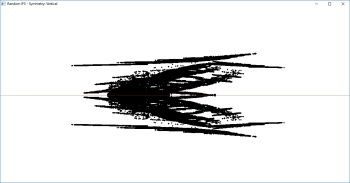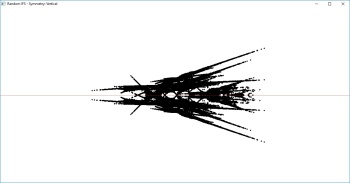However, it sometimes generates rather creepy stuff as well: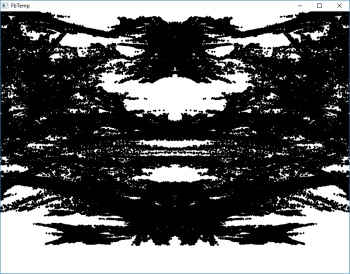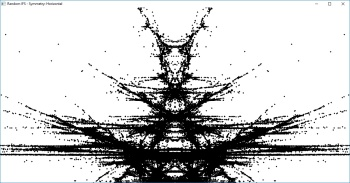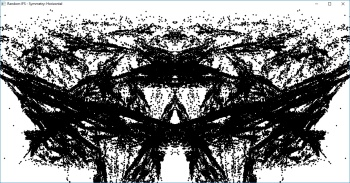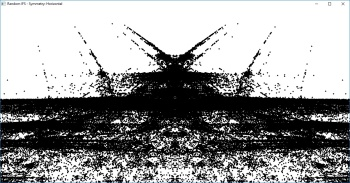That have a nice, giger-esque feel, no? It's not for the faint of hearth, though.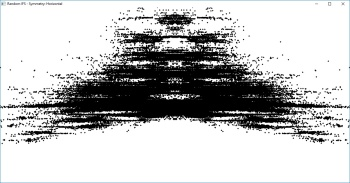(boy, do I see Bob Marley up there? This is really starting to give me the creeps)
paul doe
Posts: 915
Joined: Jul 25, 2017 17:22
Location: Argentina

### Re: Struggling with classes / types

badidea wrote:...I'll try some other stuff...

Hey, mate, did you had a look at this?

AI Block Battle

Since you've coded most of what you'll need for this one, you can use it as a base to practice and integrate more esoteric programming techniques ;)
dafhi
Posts: 1238
Joined: Jun 04, 2005 9:51

### Re: Struggling with classes / types

paul doe wrote:
dafhi wrote:infinite 2d starfield. need a reversible generator for that but this whole randomness thing is interesting

Interesting from which point of view? Artistic, mathematical, content generation?

artistic, yes. there's something therapeutic the bits
math, yep.

i need to get a grip on modulus, GCF, LCD, stuff like that, to one day do insta-calcs
have you ever seen fractint's ridiculous speed with large iter max?
I would say it's the same idea but with complex numbers you never know. cheeky bast***s

content gen - yep. i dont know GL but one day ..

paul doe wrote:I was coding an IFS generator to create concepts that could be useful in another thread. It generates interesting and cool stuff (very useful brainstorming!)
nice! noticing the anti-aliased goodness.

dark themes
1. never liked dark stuff
2. i have spiritual energy issues

if i was to use your template design game loop, where would i declare sprites?
I'll consult the metaballs demo
paul doe
Posts: 915
Joined: Jul 25, 2017 17:22
Location: Argentina

### Re: Struggling with classes / types

dafhi wrote:I'll consult the metaballs demo

And also see the animation class demo, which has a better treatment of data.
But I don't think I follow. What do you mean by 'declaring'? Global data (such as sprites, textures, et al) is declared at the global level, of course...
dafhi
Posts: 1238
Joined: Jun 04, 2005 9:51

### Re: Struggling with classes / types

the animation and shaders demos crash. i redownloaded animation and same. i can still look at the source. really dont want to use globals and was wondering if there was another option besides adding stuff to MyGameLoop (cause i dont want to mess up your class with unintentional noobie stuff)

i guess i'll just mess it up